imager contains a large array of functions for working with image data, with most of these functions coming from the CImg library by David Tschumperlé. This vignette is just a short tutorial, you’ll find more information and examples on the website. Each function in the package is documented and comes with examples, so have a look at package documentation as well.

imager comes with an example picture of boats. Let’s have a look:

``````library(imager)
plot(boats)``````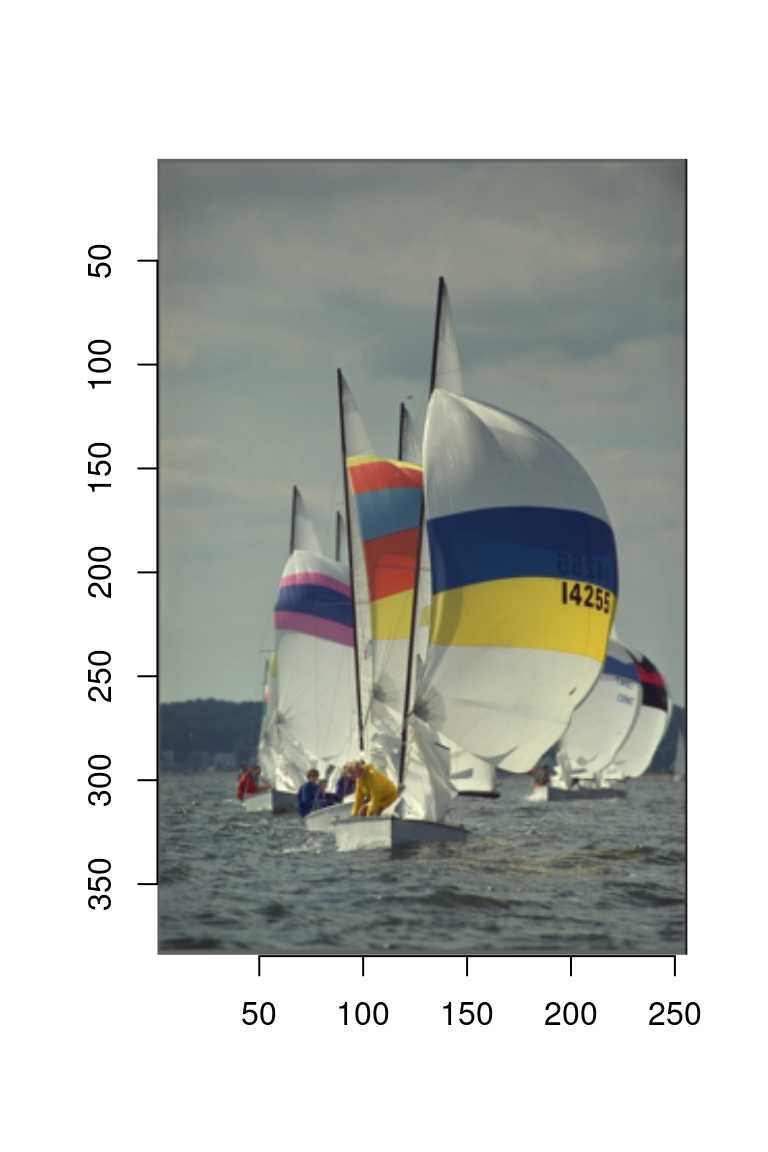Note the y axis running downwards: the origin is at the top-left corner, which is the traditional coordinate system for images. imager uses this coordinate system consistently. Image data has class “cimg”:

``class(boats)``
`` "cimg"         "imager_array" "numeric"     ``

and we can get some basic info by typing:

``boats``
``Image. Width: 256 pix Height: 384 pix Depth: 1 Colour channels: 3 ``

Width and height should be self-explanatory. Depth is how many frames the image has: if depth > 1 then the image is actually a video. Boats has three colour channels, the usual RGB. A grayscale version of boats would have only one:

``grayscale(boats)``
``Image. Width: 256 pix Height: 384 pix Depth: 1 Colour channels: 1 ``

An object of class cimg is actually just a thin interface over a regular 4D array:

``dim(boats)``
`` 256 384   1   3``

We’ll see below how images are stored exactly. For most intents and purposes, they behave like regular arrays, meaning the usual arithmetic operations work:

``log(boats)+3*sqrt(boats)``
``Image. Width: 256 pix Height: 384 pix Depth: 1 Colour channels: 3 ``
``mean(boats)``
`` 0.5089061``
``sd(boats)``
`` 0.144797``

Now you might wonder why the following two images look exactly the same:

``````layout(t(1:2))
plot(boats)
plot(boats/2)``````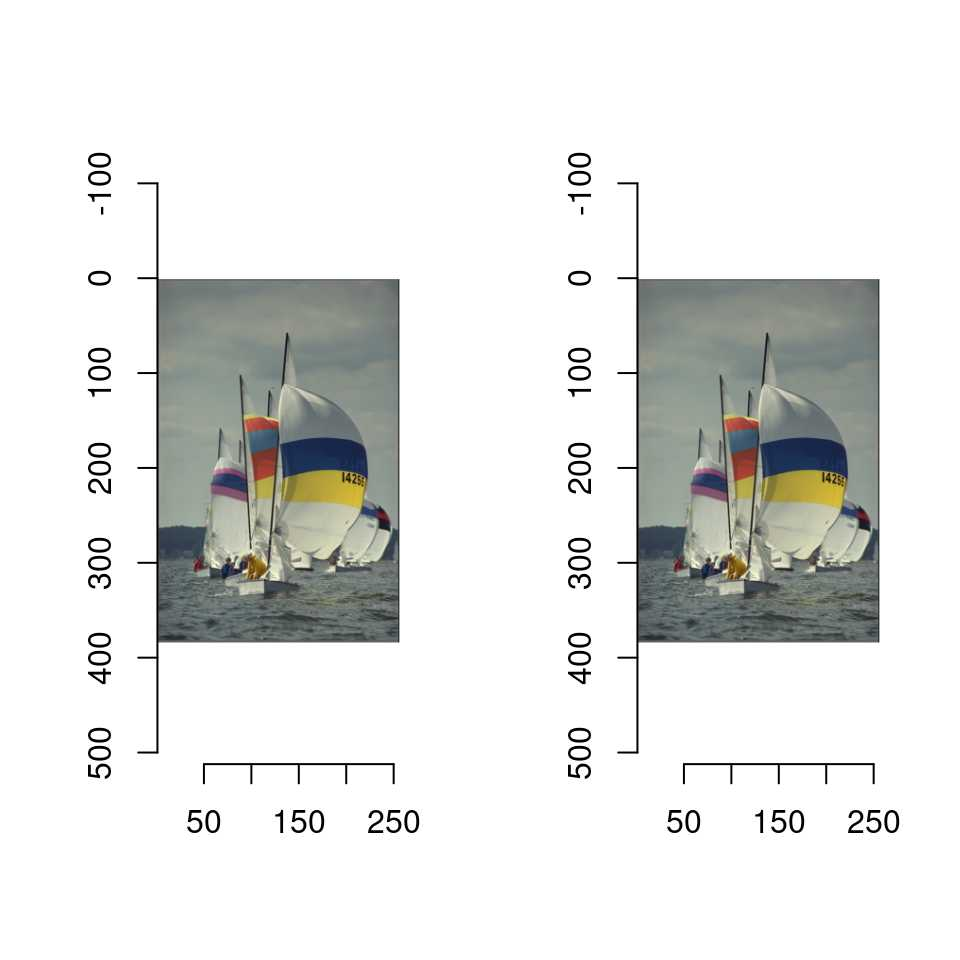That’s because the `plot` function automatically rescales the image data so that the whole range of colour values is used. There are two reasons why that’s the default behaviour:

1. There’s no agreed-upon standard for how RGB values should be scaled. Some software, like CImg, uses a range of 0-255 (dark to light), other, like R’s `rgb` function, uses a 0-1 range.
2. Often it’s just more convenient to work with a zero-mean image, which means having negative values.

If you don’t want imager to rescale the colours automatically, set rescale to FALSE, but now imager will want values that are in the \([0,1]\) range.

``````layout(t(1:2))
plot(boats,rescale=FALSE)
plot(boats/2,rescale=FALSE)``````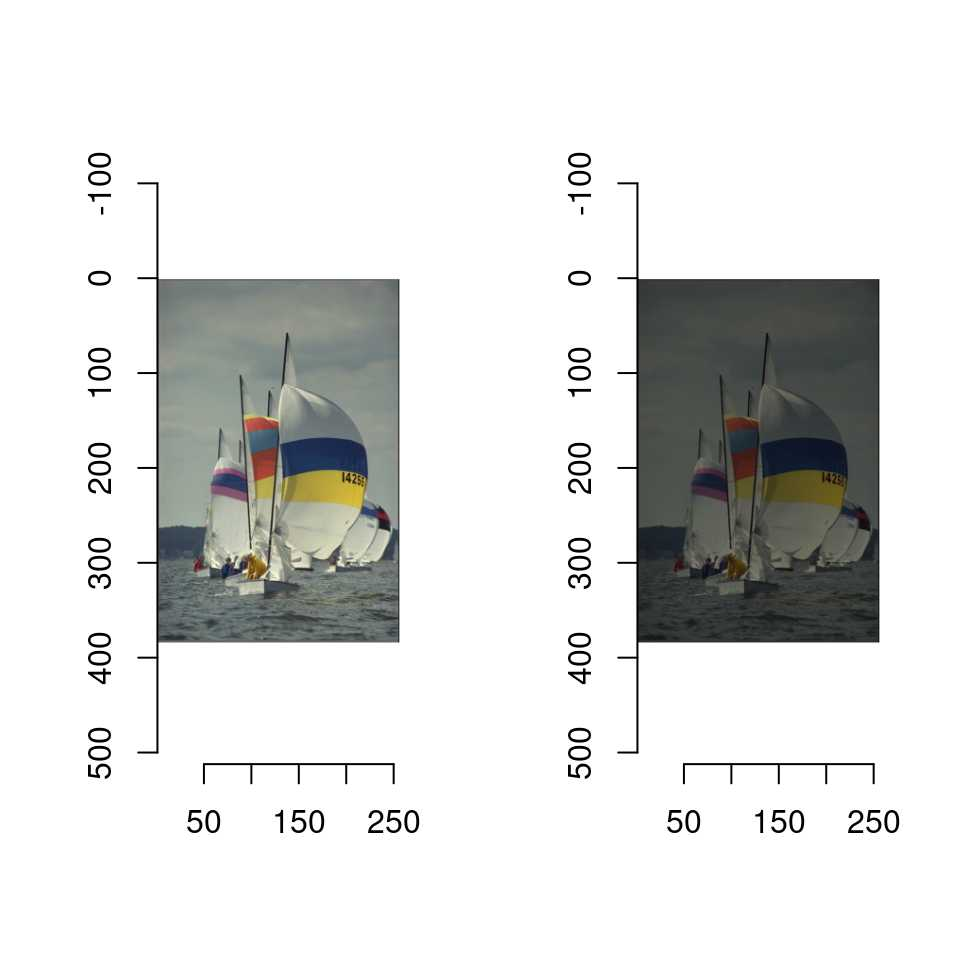If you’d like tighter control over how imager converts pixel values into colours, you can specify a colour scale. R likes its colours defined as hex codes, like so:

``rgb(0,1,0)``
`` "#00FF00"``

The function `rgb` is a colour scale, i.e., it takes pixel values and returns colours. We can define an alternative colour scale that swaps the red and green values:

``````cscale <- function(r,g,b) rgb(g,r,b)
plot(boats,colourscale=cscale,rescale=FALSE)``````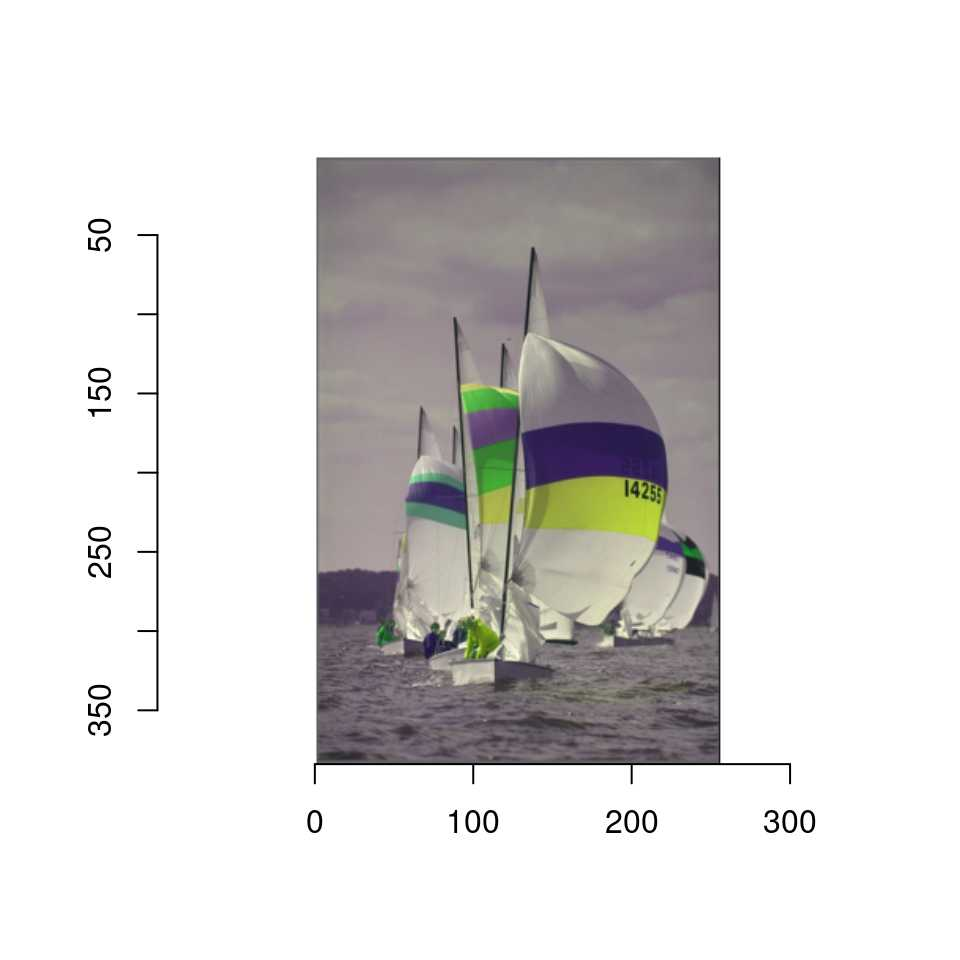In grayscale images pixels have only one value, so that the colour map is simpler: it takes a single value and returns a colour. In the next example we convert the image to grayscale

``````#Map grayscale values to blue
cscale <- function(v) rgb(0,0,v)
grayscale(boats) %>% plot(colourscale=cscale,rescale=FALSE)``````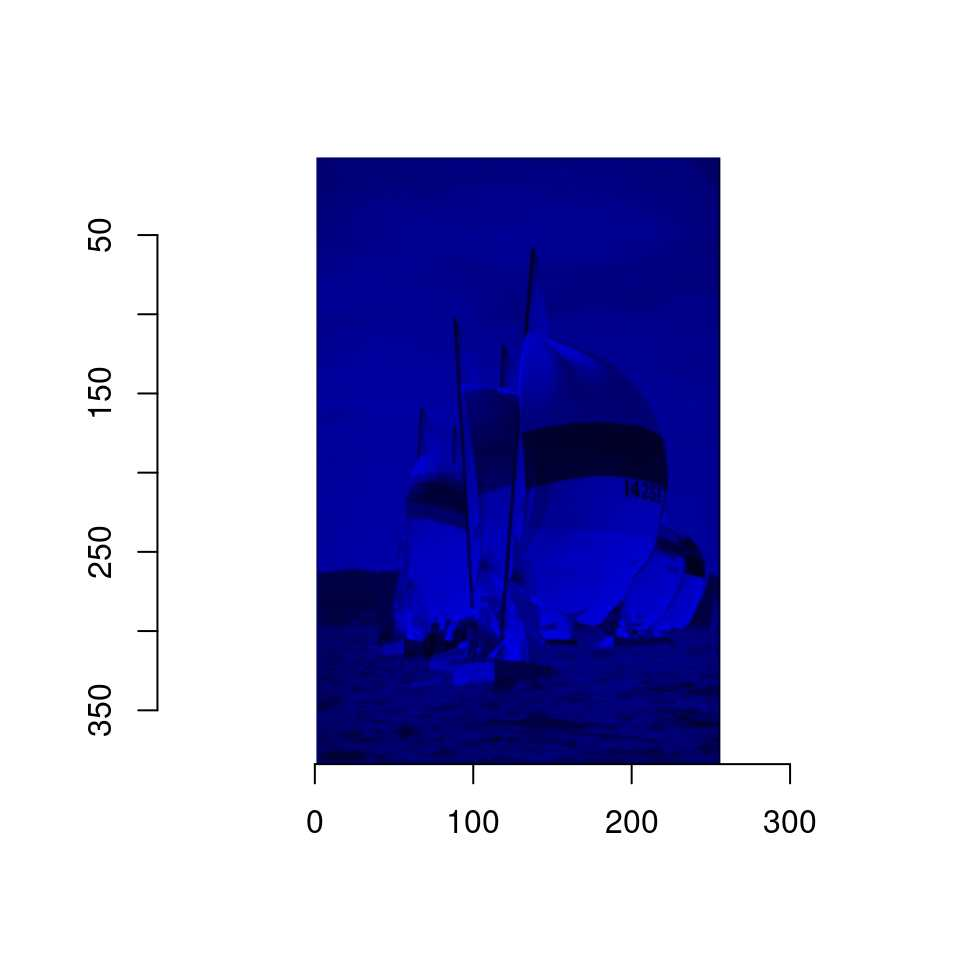The scales package has a few handy functions for creating colour scales, for example by interpolating a gradient:

``````cscale <- scales::gradient_n_pal(c("red","purple","lightblue"),c(0,.5,1))
#cscale is now a function returning colour codes
cscale(0)``````
`` "#FF0000"``
``grayscale(boats) %>% plot(colourscale=cscale,rescale=FALSE)``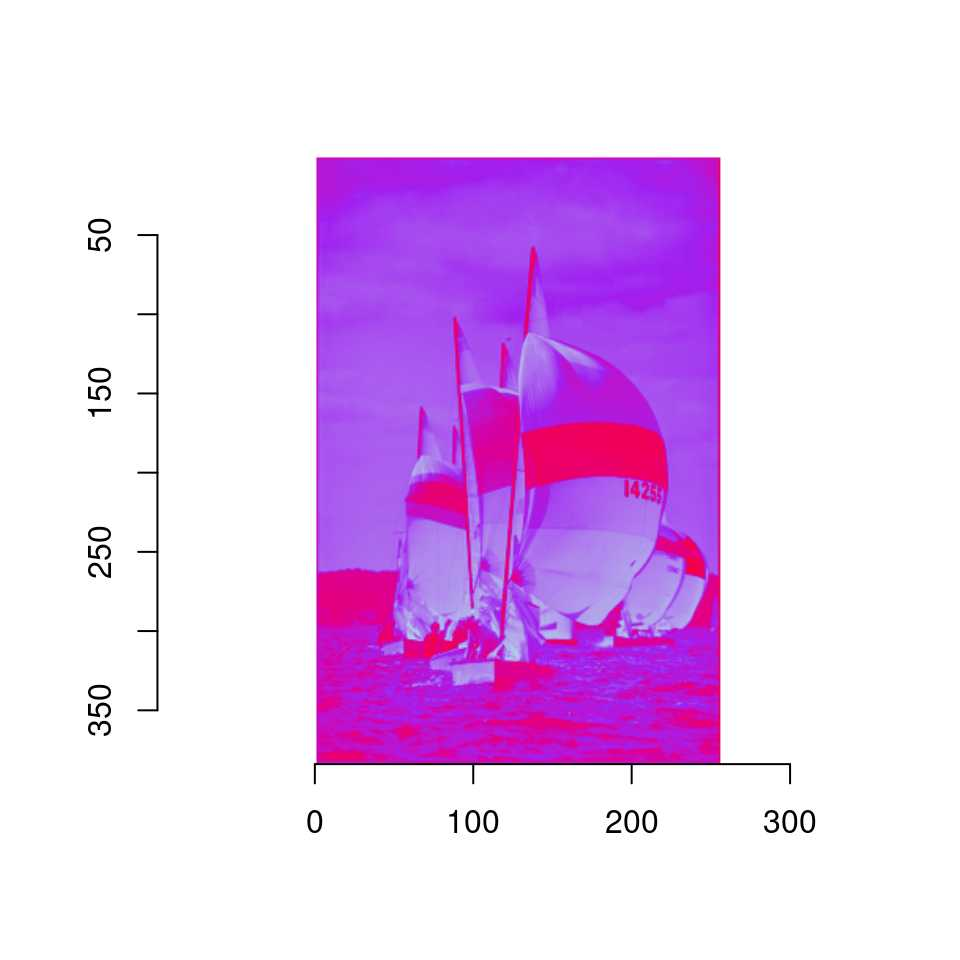See the documentation for `plot.cimg` and `as.raster.cimg` for more information and examples.

The next thing you’ll probably want to be doing is to load an image, which can be done using load.image. imager ships with another example image, which is stored somewhere in your R library. We find out where using `system.file`

``fpath <- system.file('extdata/parrots.png',package='imager')``

``````parrots <- load.image(fpath)
plot(parrots)``````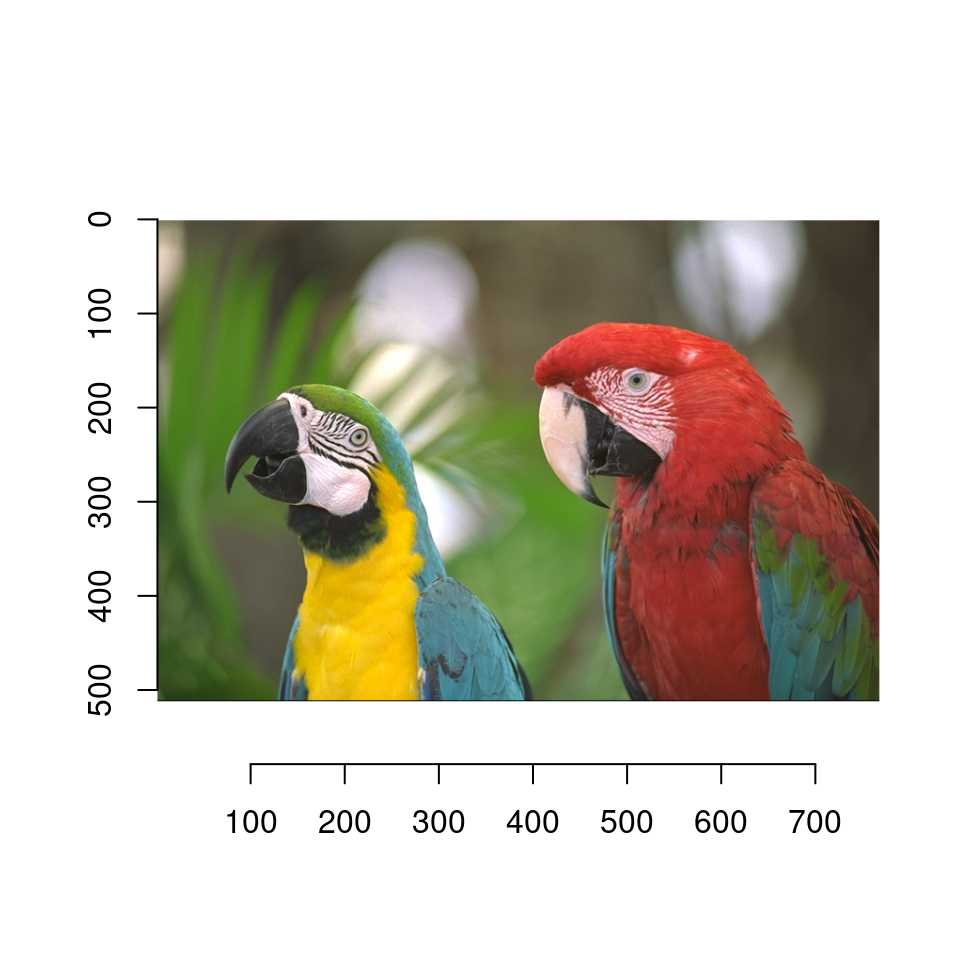imager supports JPEG, PNG, TIFF and BMP natively - for other formats you’ll need to install ImageMagick.

# 2 Example 1: Histogram equalisation

Histogram equalisation is a textbook example of a contrast-enhancing filter. It’s also a good topic for an introduction to what you can do with imager.

Image histograms are just histogram of pixel values, which are of course pretty easy to obtain in R:

``grayscale(boats) %>% hist(main="Luminance values in boats picture")``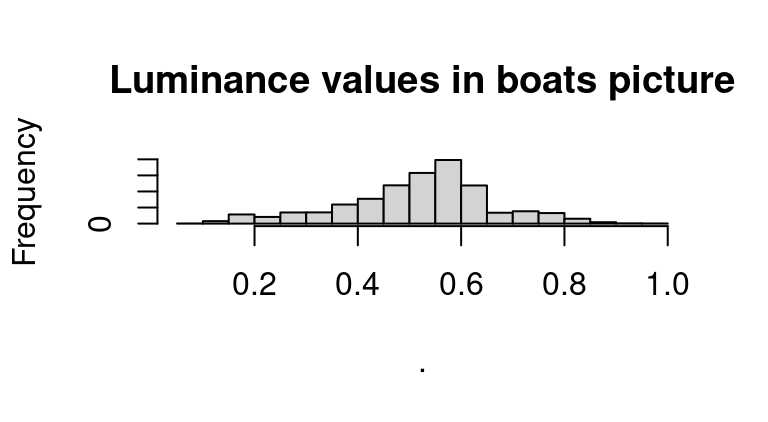Since images are stored essentially as arrays, here we’re just using R’s regular hist function, which treats our array as a vector of values. If we wanted to look only at the red channel, we could use:

``R(boats) %>% hist(main="Red channel values in boats picture")``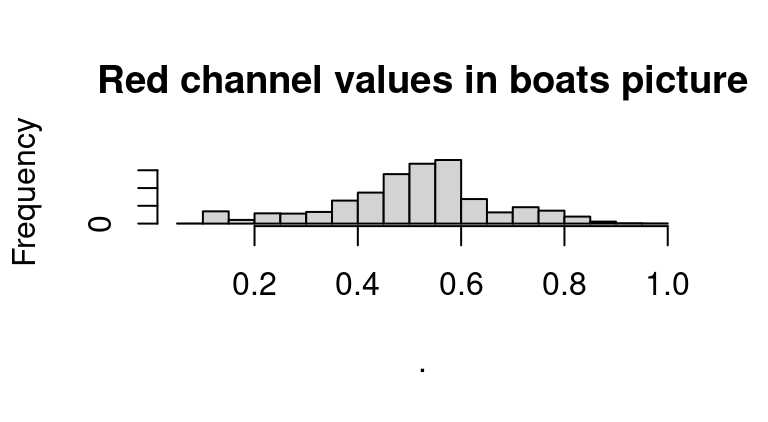``````#Equivalently:
#channel(boats,1) %>% hist(main="Red channel values in boats picture")``````

Another approach is to turn the image into a `data.frame`, and use `ggplot` to view all channels at once:

``````library(ggplot2)
library(dplyr)
bdf <- as.data.frame(boats)
``````  x y cc     value
``````bdf <- mutate(bdf,channel=factor(cc,labels=c('R','G','B')))